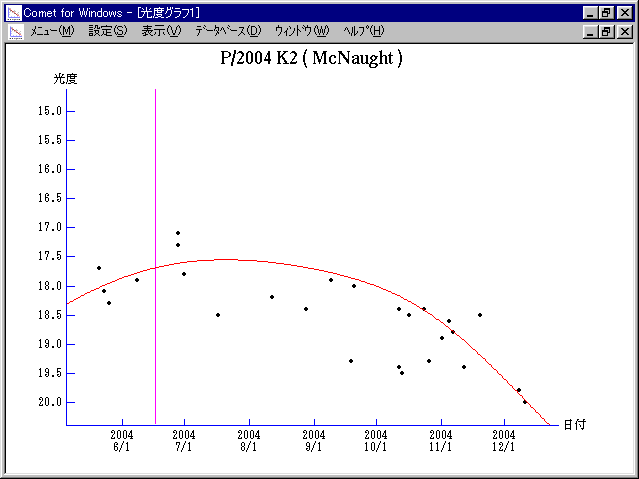# \$B%^%/%N!<%HWB@1(B

220P/McNaught (2004)

 English version Home page Updated on May 8, 2009###\$B%W%m%U%#!<%k(B

 \$BId9f(B 220P/2004 K2 \$BH/8+F|(B 2004\$BG/(B5\$B7n(B20\$BF|(B \$BH/8+8wEY(B 17.7\$BEy(B \$BH/8+ R. H. McNaught (Siding Spring)

###\$B50F;MWAG(B

```                    Epoch = 2004 June  4.0 TT
T = 2004 June 16.81457 TT        Peri. = 180.77298
e = 0.5012692                    Node  = 150.12399 2000.0
q = 1.5544515 AU                 Incl. =   8.12289
a =  3.1168146 AU   n = 0.17911734   P =   5.503 years
```

###\$B@1?^(B###\$B8wEYJQ2=(B

```        m1 = 14.0 + 5 log\$B&\$(B + 15.0 log r
```##### \$B50F;MWAG\$O!"(BCBET 1790\$B\$K7G:\\$5\$l\$?\$b\$N\$G\$9!#(B \$B@1?^\$O(B StellaNavigator Ver.2.0 for Windows (\$B%"%9%H%m%"!<%D(B\$BJTCx(B / \$B%"%9%-!<=PHG6I4)(B) \$B\$G:n@.\$7\$?\$b\$N\$G\$9!#(B \$B8wEY%0%i%U\$O(BComet for Windows\$B\$G:n@.\$7\$?\$b\$N\$G\$9!#(B